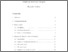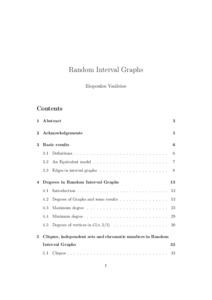# Random Interval Graphs

Iliopoulos, Vasileios (2005) Random Interval Graphs. Masters thesis, University of Essex.Preview
Text
M.Sc. thesis.pdf

Download (303kB) | Preview

## Abstract

In this thesis, which is supervised by Dr. David Penman, we examine random interval graphs. Recall that such a graph is defined by letting \$X_{1},\ldots X_{n},Y_{1},\ldots Y_{n}\$ be \$2n\$ independent random variables, with uniform distribution on \$[0,1]\$. We then say that the \$i\$th of the \$n\$ vertices is the interval \$[X_{i},Y_{i}]\$ if \$X_{i}<Y_{i}\$ and the interval \$[Y_{i},X_{i}]\$ if \$Y_{i}<X_{i}\$. We then say that two vertices are adjacent if and only if the corresponding intervals intersect. We recall from our MA902 essay that fact that in such a graph, each edge arises with probability \$2/3\$, and use this fact to obtain estimates of the number of edges. Next, we turn to how these edges are spread out, seeing that (for example) the range of degrees for the vertices is much larger than classically, by use of an interesting geometrical lemma. We further investigate the maximum degree, showing it is always very close to the maximum possible value \$(n-1)\$, and the striking result that it is equal to \$(n-1)\$ with probability exactly \$2/3\$. We also recall a result on the minimum degree, and contrast all these results with the much narrower range of values obtained in the alternative \lq comparable\rq\, model \$G(n,2/3)\$ (defined later). We then study clique numbers, chromatic numbers and independence numbers in the Random Interval Graphs, presenting (for example) a result on independence numbers which is proved by considering the largest chain in the associated interval order. Last, we make some brief remarks about other ways to define random interval graphs, and extensions of random interval graphs, including random dot product graphs and other ways to define random interval graphs. We also discuss some areas these ideas should be usable in. We close with a summary and some comments.

Item Type: Thesis (Masters) Q Science > QA Mathematics Faculty of Science and Health > Mathematical Sciences, Department of Iliopoulos Vasileios 23 Jul 2015 09:19 23 Jul 2015 09:19 http://repository.essex.ac.uk/id/eprint/14414

### Actions (login required)View Item